back

An Investor can use options to achieve a number of different things depending on the strategy the investor employs. Novice option traders will be allowed to buy calls and puts, to anticipate rising as well as falling markets.

The long call option strategy is the most basic option trading strategy whereby the options trader buys call options with the belief that the price of the stock will rise significantly beyond the strike price before the expiration date.

#### Leverage:

Compared to buying the underlying outright, the call option buyer is able to gain leverage since the lower priced calls appreciate in value faster percentage-wise for every point rise in the price of the underlying.

However, call options have a limited lifespan. If the underlying stock price does not move above the strike price before the option expiration date, the call option will expire worthless.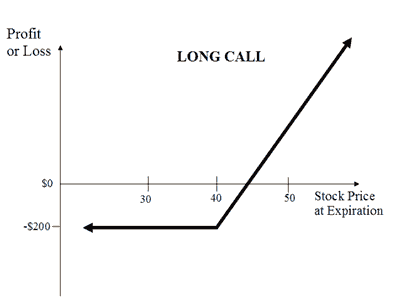#### Unlimited profit potential

Since there can be no limit as to how high the stock price can be at expiration date, there is no limit to the maximum profit possible when implementing the long call option strategy.

The formula for calculating profit is given below:

• Maximum profit = Unlimited
• Profit Achieved When Price of Underlying >=Strike Price of Long Call + Premium Paid
• Profit = Price of underlying – Strike Price of Long Call – Premium Paid

#### Limited risk

Risk for the long call options strategy is limited to the price paid for the call option no matter how low the stock price is trading on expiration date.

The formula for calculating maximum loss is given below:

• Max Loss = Premium Paid + Commissions Paid
• Max Loss Occurs when Price of Underlying <= Strike Price of Long Call

#### Breakeven point

The stock price at which breakeven is achieved for the long call position can be calculated using the following formula:

• Breakeven Point = Strike Price of Long Call + Premium Paid

The bear put spread option strategy is employed when the options trader thinks that the price of the underlying asset will go down moderately in the near term.

Bear put spreads can be implemented by buying a higher striking in-the-money put option and selling a lower striking out-of-the-money put option of the same underlying security with the same expiration date.

#### Sell 1 OTM Put

By shorting the out-of-the-money put, the options trader reduces the cost of establishing the bearish position but forgoes the chance of making a large profit in the event that the underlying asset price plummets.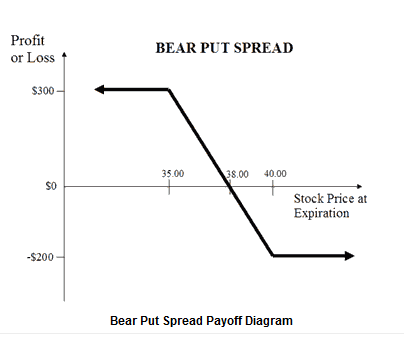#### Limited downside profit

To reach maximum profit, the underlying needs to close below the strike price of the out-of-the-money put on the expiration date. Both options expire in the money but the higher strike put that was purchased will have higher intrinsic value than the lower strike put that was sold. Thus, maximum profit for the bear put spread option strategy is equal to the difference in strike price minus the debit taken when the position was entered.

The formula for calculating maximum profit is given below:

• Max profit = Strike Price of Long Put – Strike Price of Short Put – Net Premium Paid – Commissions
• Max Profit Achieved When Price of Underlying <= strike price of Short Put

#### Limited upside risk

If the stock price rise above the in-the-money put option strike price at the expiration date, then the bear put spread strategy suffers a maximum loss equal to the debit taken when putting on the trade.

• Max Loss = Net Premium Paid + Commissions Paid
• Max Loss Occurs when Price of Underlying >= Strike Price of Long Put

#### Breakeven point

The stock price at which breakeven is achieved for the bear put spread position can be calculated using the following formula:

• Breakeven Point = Strike Price of Long Put – Net Premium Paid

The bull call spread option strategy is employed when the options trader thinks that the price of the underlying asset will go up moderately in the near term. Bull call spreads can be implemented by buying an at-the-money call option while simultaneously writing a higher striking out-of-the-money call option of the same underlying and the same expiration month.

#### Sell 1 OTM Call

By shorting the out-of-the-money call, the options trader reduces the cost of establishing the bullish position but forgoes the chance of making a large profit in the event that the underlying asset price skyrockets.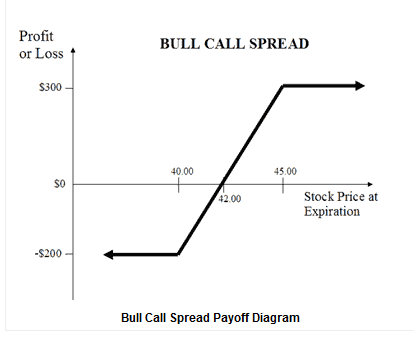#### Limited upside profits

Maximum gain is reached for the bull call spread options strategy when the underlying price moves above the higher strike price of the two calls and its equal to the difference between the price strike of the two call options minus the initial debit taken to enter the position.

The formula for calculating maximum profit is given below:

• Max Profit = Strike Price of Short Call – Strike Price of Long Call – Net Premium Paid – Commissions Paid
• Max Profit Achieved When Price of Underlying >= Strike Price of Short Call

#### Limited downside risk

The bull call spread strategy will result in a loss if the underlying price declines at expiration. Maximum loss cannot be more than the initial debit taken to enter the spread position.

The formula for calculating maximum loss is given below:

• Max Loss = Net Premium Paid + Commissions Paid
• Max Loss Occurs When Price of Underlying <= Strike Price of Long Call

#### Breakeven point

The stock price at which breakeven is achieved for the bull call spread position can be calculated using the following formula:

• Breakeven Point = Strike Price of Long Call + Net Premium Paid

The covered call is a strategy in options trading whereby call options are written against a holding of the underlying security.

#### Sell 1 Call

Using the covered call option strategy, the investor gets to earn a premium writing calls while at the same time appreciate all benefits of underlying stock ownership, such as dividends and voting rights, unless he is assigned an exercise notice on the written call and is obliged to sell his shares.

However, the profit potential of covered call writing is limited as the investor has, in return for the premium, given up the chance to fully profit from a substantial rise in the price of the underlying asset.

#### Out-of-the-money covered call

This is a covered call strategy where the moderately bullish investor sells out-of-the-money calls against a holding of the underlying shares. The OTM covered call is a popular strategy as the investor gets to collect premium while being able to enjoy capital gains if the underlying stock rallies.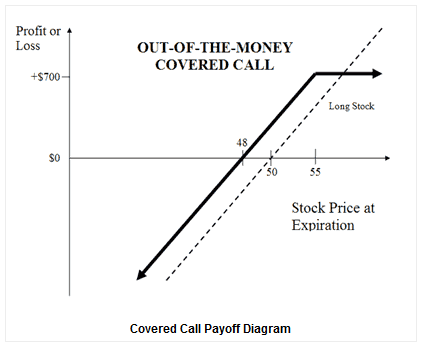#### Limited profit potential

In addition to the premium received for writing the call, the OTM covered call strategy’s profit also includes gain if the underlying stock price rises, up to the strike price of the call option sold.

The formula for calculating maximum profit is given below:

• Max Profit = Premium received – Purchase Price of the Underlying + Strike Price of Short Call – Commissions Paid
• Max Profit Achieved when Price of Underlying>= Strike Price of Short Call

#### Unlimited loss potential

Potential losses for this strategy can be very large and occurs when the price of the stock falls. However, this risk is no different than that which the typical stock owner is exposed to. In fact, the covered call writer’s loss is cushioned slightly by the premiums received for writing the calls.

The formula for calculating loss is given below:

• Maximum loss = Unlimited
• Loss Occurs When Price of Underlying < purchase price of underlying – Premium received
• Loss = Purchase Price of Underlying – Price of Underlying – Max Profit + Commissions Paid

#### Breakeven point

The stock price at which breakeven is achieved for the covered call (OTM) position can be calculated using the following formula:

The long put option strategy is a basic strategy in options trading where the investor buys put options with the belief that the price of the underlying will go significantly below the strike price before the expiration date.

Compared to short-selling the underlying, it is more convenient to bet against an underlying by purchasing put options. The risk is capped to the premium paid for the put options, as opposed to unlimited risk when short-selling the underlying outright.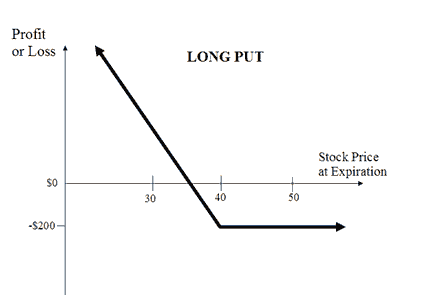#### Limited potential

Since the stock price, in theory, can reach zero at the expiration date, the maximum profit possible when using the long put strategy is limited to the strike price of the purchased put less the price paid for the option.

The formula for calculating profit is given below:

• Maximum Profit = Unlimited
• Profit Achieved when Price of Underlying = 0
• Profit = Strike Price of Long Put – Premium Paid Limited risk

Risk for implementing the long put strategy is limited to the price paid for the put option no matter how high the underlying price is trading on expiration date.

The formula for calculating maximum loss is given below:

• Max Loss = Premium Paid + Commissions Paid
• Max Loss Occurs When Price of Underlying >= Strike Price of Long Put

#### Breakeven point

The underlying price at which breakeven is achieved for the long put position can be calculated using the following formula:

• Breakeven Point = Strike Price of Long Put – Premium Paid

The long straddle is a neutral strategy in options trading that involves the simultaneous buying of a put and a call of the same underlying asset, strike price and expiration date.

Long straddle options are unlimited profit/limited risk options trading strategies that are used when the options trader thinks that the underlying asset will experience significant volatility in the near term.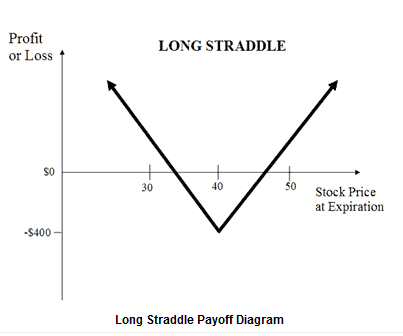#### Unlimited profit potential

A large gain for the long straddle option strategy is attainable when the underlying stock price makes a very strong move either upwards or downwards at expiration.

The formula for calculating profit is given below:

• Maximum Profit Unlimited
• Profit Achieved When Price of Underlying > Strike Price of Long Call + Net Premium Paid or Price of Underlying < strike  price  of  long put  – net
• Profit = Price of Underlying – Strike Price of Long Call – Net Premium Paid or Strike Price of Long Put – Price of Underlying – Net Premium Paid

#### Limited risk

Maximum loss for the long straddle options strategy is hit when the underlying stock price on expiration date is trading between the strike prices of the options bought. At this price, both options expire worthless and the options trader loses the entire initial debit taken to enter the trade.

The formula for calculating maximum loss is given below:

• Max Loss = Net Premium Paid + Commissions Paid
• Max loss occurs when price of underlying is equal to the strike price

#### Breakeven points

There are two breakeven points for the long straddle position. The breakeven points can be calculated using the following formulae:

• Upper Breakeven Point = Strike Price of Long Call + Net Premium Paid
• Lower Breakeven Point = Strike Price of Long Put – Net Premium Paid

The long strangle, is a neutral strategy in options trading that involves the simultaneous buying of a slightly out-of-the-money put and a slightly out-of-the-money call of the same underlying asset and expiration date.

#### Long strangle construction

The long options strangle is an unlimited profit/limited risk strategy used when the options trader thinks that the underlying stock will experience significant volatility in the near term. Long strangles are debit spreads as a net debit is taken to enter the trade.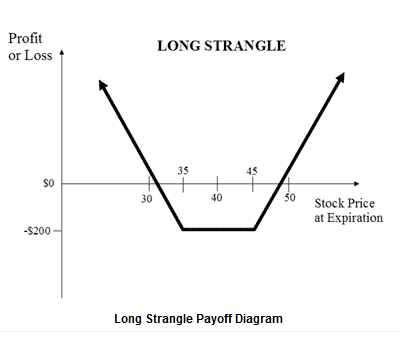#### Unlimited profit potential

A large gain for the long straddle option strategy is attainable when the underlying stock price makes a very strong move either upwards or downwards at expiration.

The formula for calculating profit is given below:

• Maximum Profit Unlimited
• Profit Achieved When Price of Underlying > Strike Price of Long Call + Net Premium Paid or Price of Underlying < strike Price of Long Put – Net Premium Paid
• Profit = Price of Underlying – Strike Price of Long Call – Net Premium Paid or Strike Price of Long Put – Price of Underlying – Net Premium Paid

#### Limited risk

Maximum loss for the long strangle options strategy is hit when the underlying stock price on expiration date is trading between the strike prices of the options bought. At this price, both options expire worthless and the options trader loses the entire initial debit taken to enter the trade.

The formula for calculating maximum loss is given below:

• Max Loss = Net Premium Paid + Commissions Paid
• Max Loss Occurs When Price of Underlying is in between Strike Price of Long Call and Strike Price of Long Put

#### Breakeven points

There are two breakeven points for the long strangle position. The breakeven points can be calculated using the following formulae:

• Upper Breakeven Point = Strike Price of Long Call + Net Premium Paid
• Lower Breakeven Point = Strike Price of Long Put – Net Premium Paid

The naked call write is a risky options trading strategy where the options trader sells calls against stock which he does not own. Also known as uncovered call writing.

The out-of-the-money naked call strategy involves writing out-of-the money call options without owning the underlying stock. It is a premium collection options strategy employed when one is neutral to mildly bearish on the underlying.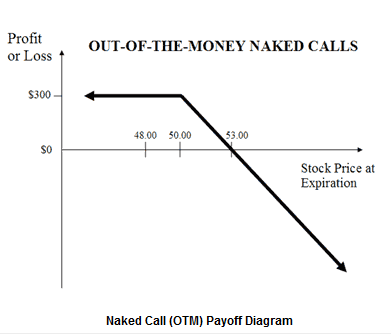#### Limited profit potential

Maximum gain is limited and is equal to the premium collected for selling the call options.

The formula for calculating maximum profit is given below:

• Max Profit Achieved When Price of Underlying <= Strike Price of Short Call

#### Unlimited loss potential

If the underlying price goes up dramatically at expiration, the out-of-the-money naked call writer will be required to satisfy the options requirements to sell the obligated underlying to the options holder at the lower price, buying the underlying at the open market price. Since there is no limit to how high the underlying price can be at expiration, maximum potential losses for writing out-of-the-money naked calls is therefore theoretically unlimited.

The formula for calculating loss is given below:

• Maximum Loss = Unlimited
• Loss Occurs When Price of Underlying > Strike Price of Short Call + Premium Received
• Loss = Price of Underlying – Strike price of Short Call – Premium Received + Commissions Paid

#### Breakeven point

The stock price at which break-even is achieved for the naked call (OTM) position can be calculated using the following formula:

A risk reversal, or collar,is an option strategy that is constructed by holding shares of the underlying stock while simultaneously buying protective puts and selling call options against the holding. The puts and the calls are both out-of-the-money options having the same expiration month and must be equal in number of contracts.

#### Sell 1 OTM Call

Technically, the risk reversal strategy is the equivalent of an out-of-the-money covered call strategy with the purchase of an additional protective put.

The risk reversal strategy is a good strategy to use if the options trader is writing covered call to earn premium but wishes to protect himself from an unexpected sharp drop in the price of the underlying asset.

#### Limited profit potentialThe formula for calculating maximum profit is given below:

• Max Profit = Strike Price of Short Call – Purchase Price of Underlying + Net Premium Received – Commissions Paid
• Max Profit Achieved When Price of Underlying >= Strike Price of Short Call

#### Limited risk

The formula for calculating maximum loss is given below:

• Max Loss = Purchase Price of Underlying – Strike Price of Long Put – Net Premium Received + Commissions Paid.
• Max Loss Occurs When Price of Underlying <= Strike Price of Long Put

#### Breakeven point

The stock price at which breakeven is achieved for the risk reversal strategy position can be calculated using the following formula:

• Breakeven Point = Purchase Price of Underlying + Net Premium Paid

Writing uncovered puts is an options trading strategy involving the selling of put options without shorting the obligated underlying. Also known as a naked put write or cash secured put, this is a bullish options strategy that is executed to earn a consistent profit by ongoing collection of premium.

#### Sell 1 ATM Put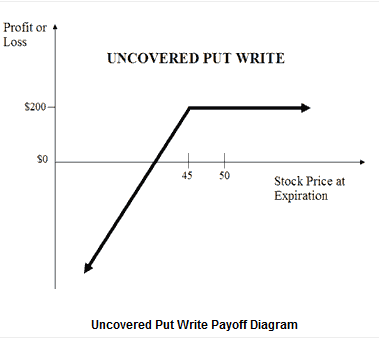#### Limited profits with no upside risk

Profit for the uncovered put write is limited to the premiums received for the options sold. The naked put writer sells slightly out-of-the-money puts month after month, collecting premiums as long as the stock price of the underlying remains above the put strike price at expiration.

• Max Profit Achieved when Price of Underlying >= Strike Price of short Put

#### Unlimited downside risk with little downside protection

While the premium collected can cushion a slight drop in the underlying price, loss resulting from a catastrophic drop in the price of the underlying can be huge.

The formula for calculating loss is given below:

• Maximum Loss = Unlimited
• Loss Occurs When Price of Underlying < Strike Price of Short Put – Premium Received
• Loss = Strike Price of Short Put – Price of Underlying – Premium received + Commissions Paid

#### Breakeven point

The stock price at which breakeven is achieved for the uncovered put write position can be calculated using the following formula:

• Breakeven Point = Strike Price of Short Put – Premium Received

The short straddle or naked straddle sale is a neutral options strategy that involves the simultaneous selling of a put and a call of the same underlying stock, strike price and expiration date.

Short straddles are limited profit/unlimited risk options trading strategies that are used when the options trader thinks that the underlying security will experience little volatility in the near term.

#### Sell 1 ATM Put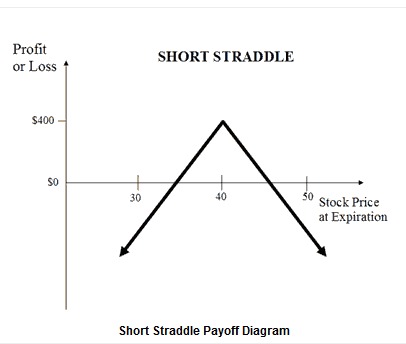#### Limited profit

Maximum profit for the short straddle is achieved when the underlying stock price on expiration date is trading at the strike price of the options sold. At this price, both options expire worthless and the options trader gets to keep the entire initial credit taken as profit.

The formula for calculating maximum profit is given below:

• Max Profit Achieved When Price of Underlying = Strike Price of Short Call/Put

#### Unlimited risk

Large losses for the short straddle can be incurred when the underlying price makes a strong move either upwards or downwards at expiration, causing the short call or the short put to expire deep in the money.

The formula for calculating loss is given below:

• Maximum Loss = Unlimited
• Loss Occurs when Price of Underlying > Strike Price of Short call + Net premium received or Price of underlying < Strike Price of Short put – Premium received.

#### Breakeven points

There are two breakeven points for the short straddle position. The breakeven points can be calculated using the following formulae:

• Upper Breakeven Point = Strike Price of Short Call + Net Premium Received
• Lower Breakeven Point = Strike Price of Short Put – Net Premium Received

The short strangle option strategy is a limited profit/unlimited risk options trading strategy that is used when the options trader thinks that the underlying stock will experience little volatility in the near term.

#### Sell 1 OTM Put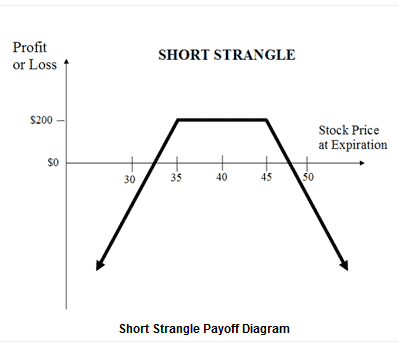#### Limited profit

Maximum profit for the short strangle occurs when the underlying stock price on expiration date is trading between the strike prices of the options sold. At this price, both options expire worthless and the options trader gets to keep the entire initial credit taken as profit.

The formula for calculating maximum profit is given below:

• Max Profit Achieved When Price of underlying is inbetween the Strike Price of the Short Call and the Strike Price of the Short Put

#### Unlimited risk

Large losses for the short strangle can be experienced when the underlying stock price makes a strong move either upwards or downwards at expiration.

The formula for calculating loss is given below:

• Maximum Loss = Unlimited
• Loss Occurs When Price of underlying > Strike Price of short Call + Net Premium Received or Price of Underlying < Strike Price of Short Put – Net Premium Received.
• Loss = Price of Underlying – strike Price of Short Call – Net Premium received or Strike Price of short Put – Price of Underlying – Net Premium Received.

#### Breakeven points

There are two breakeven points for the short strangle position. The breakeven points can be calculated using the following formulae:

• Upper Breakeven Point = Strike Price of Short Call + Net Premium Received
• Lower Breakeven Point = Strike Price of Short Put – Net Premium Received

## See all our prices

Get ultra-competitive spreads and commissions across all asset classes, and receive even better rates as your volume increases.

Opening an account takes around 5 minutes## Listed options risk warning

An option is categorised as a red product as it is considered an investment product with a high complexity and a high risk.
Danish banks are required to categorise investment products offered to retail clients depending on the product’s complexity and risk as: green, yellow or red. For further information click here

Options involve risks and are not suitable for all investors as the special risks inherent to options trading may expose investors to potentially rapid and substantial losses. Options trading privileges are subject to Saxo Bank A/S review and approval. Prior to buying or selling an option, investors must read a copy of the Characteristics & Risks of Standardized Options, also known as the options disclosure document (ODD). It explains the characteristics and risks of exchange- traded options.

Other Risks: Spreads, Straddles, and other multiple-leg option strategies can entail substantial transaction costs, including multiple commissions, which may impact any potential return.  These are advanced option strategies and often involve greater risk, and more complex risk, than basic options trades.

Exercise and assignment of options, particularly American-style, may lead to substantial losses especially if a writer of the option is "uncovered." Options that expire in-the- money are subject to automatic exercise, while options that are out-of-the-money are expired. In some cases, holders of long OTM options may decide to exercise if very close to the daily settlement underlying price, e.g. "Pin Risk."